• 1.()已知直线x+2y－3=0与圆x2+y2+x－6y+m=0相交于PQ两点，O为坐标原点，若OPOQ，则m等于(　　 )

A.3　　　　　　　　　　　　　　　 B.－3　　　　　　　　　　　　　 C.1　　　　　　　　　　　 D.－1

• 2.()中心在原点，焦点在坐标为(0，±5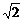)的椭圆被直线3xy－2=0截得的弦的中点的横坐标为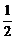，则椭圆方程为(　　 )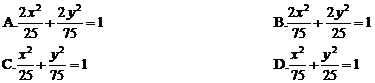• 3.()直线l的方程为y=x+3,在l上任取一点P，若过点P且以双曲线12x2－4y2=3的焦点作椭圆的焦点，那么具有最短长轴的椭圆方程为_________.

• 4.()已知圆过点P(4，－2)、Q(－1，3)两点，且在y轴上截得的线段长为4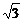，则该圆的方程为_________.

• 5.()已知椭圆的中心在坐标原点，焦点在x轴上，它的一个焦点为FM是椭圆上的任意点，|MF|的最大值和最小值的几何平均数为2，椭圆上存在着以y=x为轴的对称点M1M2，且|M1M2|=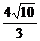，试求椭圆的方程.

• 6.()某抛物线形拱桥跨度是20米，拱高4米，在建桥时每隔4米需用一支柱支撑，求其中最长的支柱的长.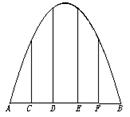• 7.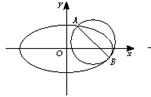()已知圆C1的方程为(x－2)2+(y－1)2=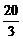,椭圆C2的方程为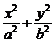=1(ab＞0)，C2的离心率为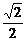，如果C1C2相交于AB两点，且线段AB恰为圆C1的直径，求直线AB的方程和椭圆C2的方程.

1.解析：设F1(－c,0)、F2(c,0)、P(x,y),则

|PF1|2+|PF2|2=2(|PO|2+|F1O|2)＜2(52+c2),

∴16+8c2＜50+2c2,∴c2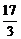,

2.解法一：设所求圆的圆心为P(a,b)，半径为r,则点Px轴、y轴的距离分别为|b|、|a|

∵圆Py轴所得弦长为2，∴r2=a2+1

r2=2b2, ∴r2=2

A(0,y1),B(0,y2)是圆与y轴的两个交点，则y1y2是方程a2+(yb)2=r2的两根，

y12=b±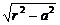x12=a±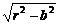a－2b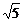d,得a2=(2b±d)2=4b2±4bd+5d2

∵方程有实根.

Δ=8(5d2－1)≥0,得5d2≥1.

dmin=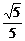,将其代入2b2±4bd+5d2+1=0,

y1y2=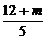,y1+y2=4.

x1x2=(3－2y1)(3－2y2)=4y1y2－6(y1+y2)+9

y1y2+x1x2=5y1y2－6(y1+y2)+9=m－3=0，故m=3.

2.解析：由题意，可设椭圆方程为：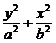=1,且a2=50+b2,

x1+x2=1可求得b2=25,a2=75.

4.解析：设所求圆的方程为(xa)2+(yb)2=r2

b2=4,设椭圆方程为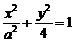①

M1(x1,y1)、M2(x2,y2),M1M2的中点为(x0,y0),

x0=(x1+x2)=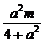,y0=－x0+m=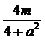.

6.解：以拱顶为原点，水平线为x轴，建立坐标系，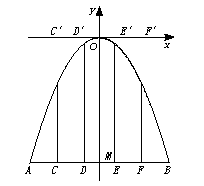(－0.16)－(－4)=3.84.故最长支柱长应为3.84米.

7.解：由e=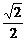,可设椭圆方程为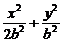=1,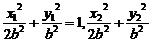=1,两式相减，得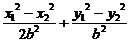=0,

Δ=24b2－72＞0,又|AB|=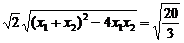,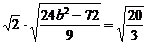，解得b2=8.